﻿ 联合安徽CORS分析郯庐断裂带南段地壳形变与断层活动特征文章快速检索 高级检索
 大地测量与地球动力学2022, Vol. 42Issue (1): 65-70  DOI: 10.14075/j.jgg.2022.01.013引用本文CHEN Hao, TAO Tingye, LI Shuiping, et al. Analysis of Crustal Deformation and Fault Activity Characteristics in the Southern Segment of Tanlu Fault Zone with AHCORS[J]. Journal of Geodesy and Geodynamics, 2022, 42(1): 65-70.Foundation support

Natural Science Foundation of Anhui Province, No.1808085MD105.

Corresponding author

TAO Tingye, PhD, associate professor, majors in GNSS algorithms and applications, lidar technology and applications, E-mail: czytty@163.com.

第一作者简介

CHEN Hao, postgraduate, majors in GNSS data processing and crustal deformation, E-mail: ch16229@126.com.

文章历史

1. 合肥工业大学土木与水利工程学院，合肥市屯溪路193号，230009

1 GNSS数据处理表 1 数据处理策略 Tab. 1 Data processing strategy图 1 郯庐断裂带南段及周边区域水平运动速度场 Fig. 1 Horizontal velocity field on the southern of the Tanlu fault and its surrounding areas表 2 欧亚框架下AHCORS参考站在NEU方向速度估值及中误差统计 Tab. 2 Velocity valuation and medium error of AHCORS in N, E and U directions in Eurasian plate
2 联合AHCORS参考站的多尺度球面小波应变场解算 2.1 多尺度球面小波基本原理

 $\begin{array}{l} {\psi _{x, a}}X' = {\lambda _a}{\left( \gamma \right)^{\frac{1}{2}}}{\rm{exp}}\left[ { - \frac{{{{\tan }^2}\left( {\frac{\gamma }{2}} \right)}}{{{a^2}}}} \right] - \\ \;\;\;\;\;\;\;\frac{1}{a}{\lambda _{{\alpha ^a}}}{\left( \gamma \right)^{1/2}}{\rm{exp}}\left[ { - \frac{{{{\tan }^2}\left( {\frac{\gamma }{2}} \right)}}{{\alpha {a^2}}}} \right] \end{array}$ (1)

2.2 多尺度球面小波估计速度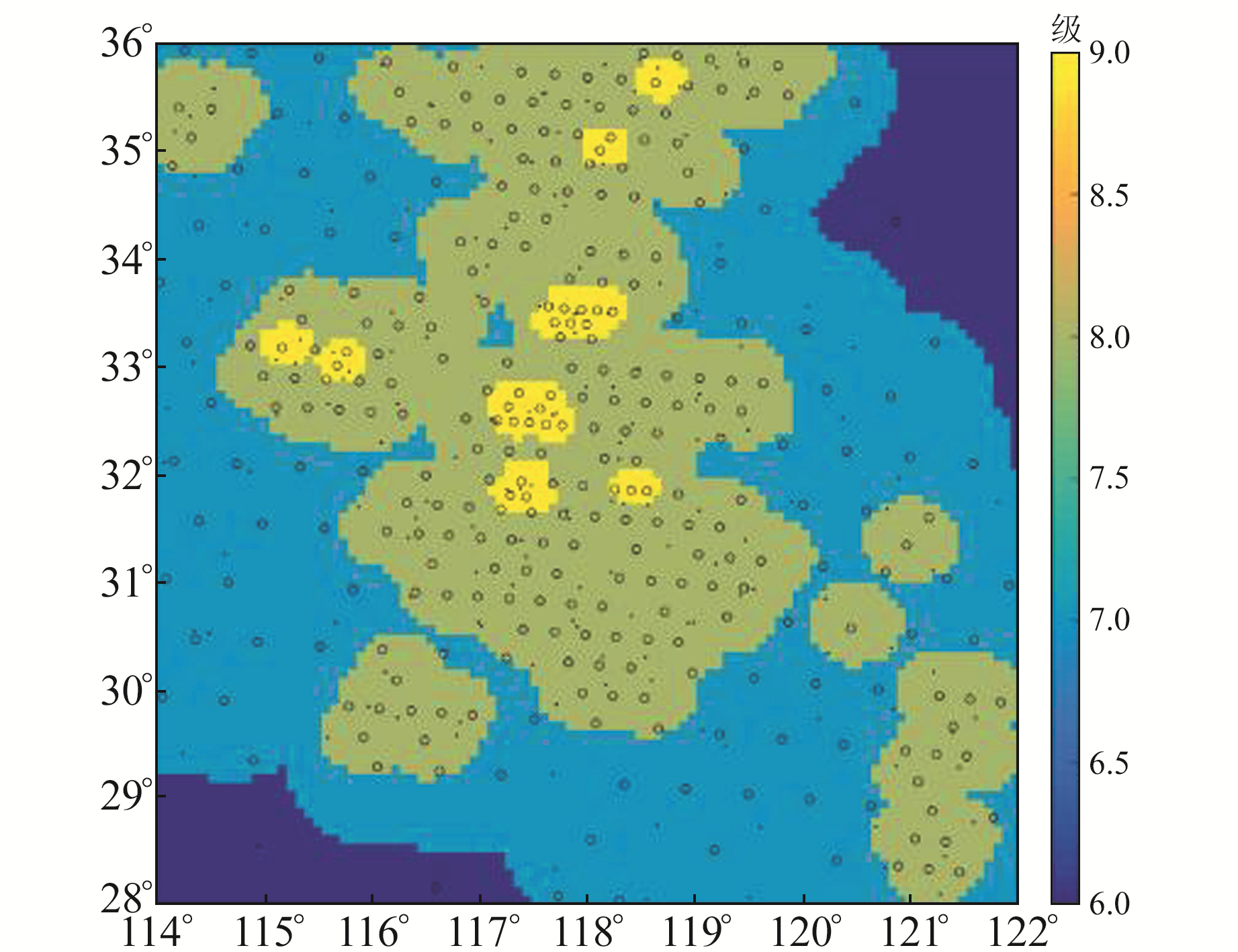图 2 由AHCORS参考站分布确定的球面小波分解尺度 Fig. 2 Spherical wavelet decomposition scale based on AHCORS distribution

 $\nu \left( {\theta , \varphi } \right) = {\nu _\theta }\left( {\theta , \varphi } \right)\hat \theta + {\nu _\varphi }\left( {\theta , \varphi } \right)\hat \varphi$ (2)

 $\nu \left( {\theta , \varphi } \right) = \mathop \sum \limits_{k = 1}^n \left[ {{a_k}{g_k}\left( {\theta , \varphi } \right)\hat \theta + {b_k}{g_k}\left( {\theta , \varphi } \right)\hat \varphi } \right]$ (3)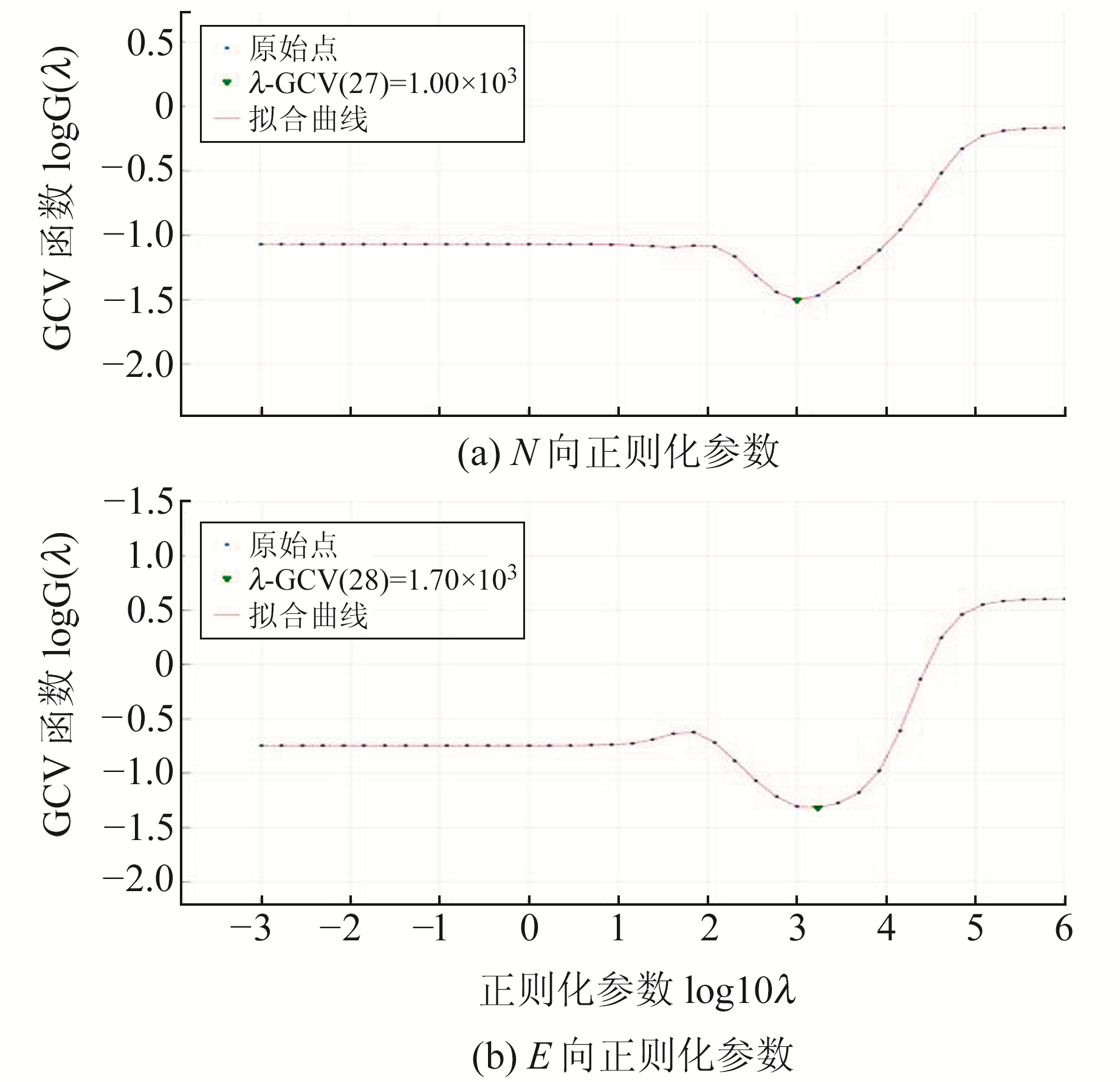图 3 正则化参数 Fig. 3 Regularization parameter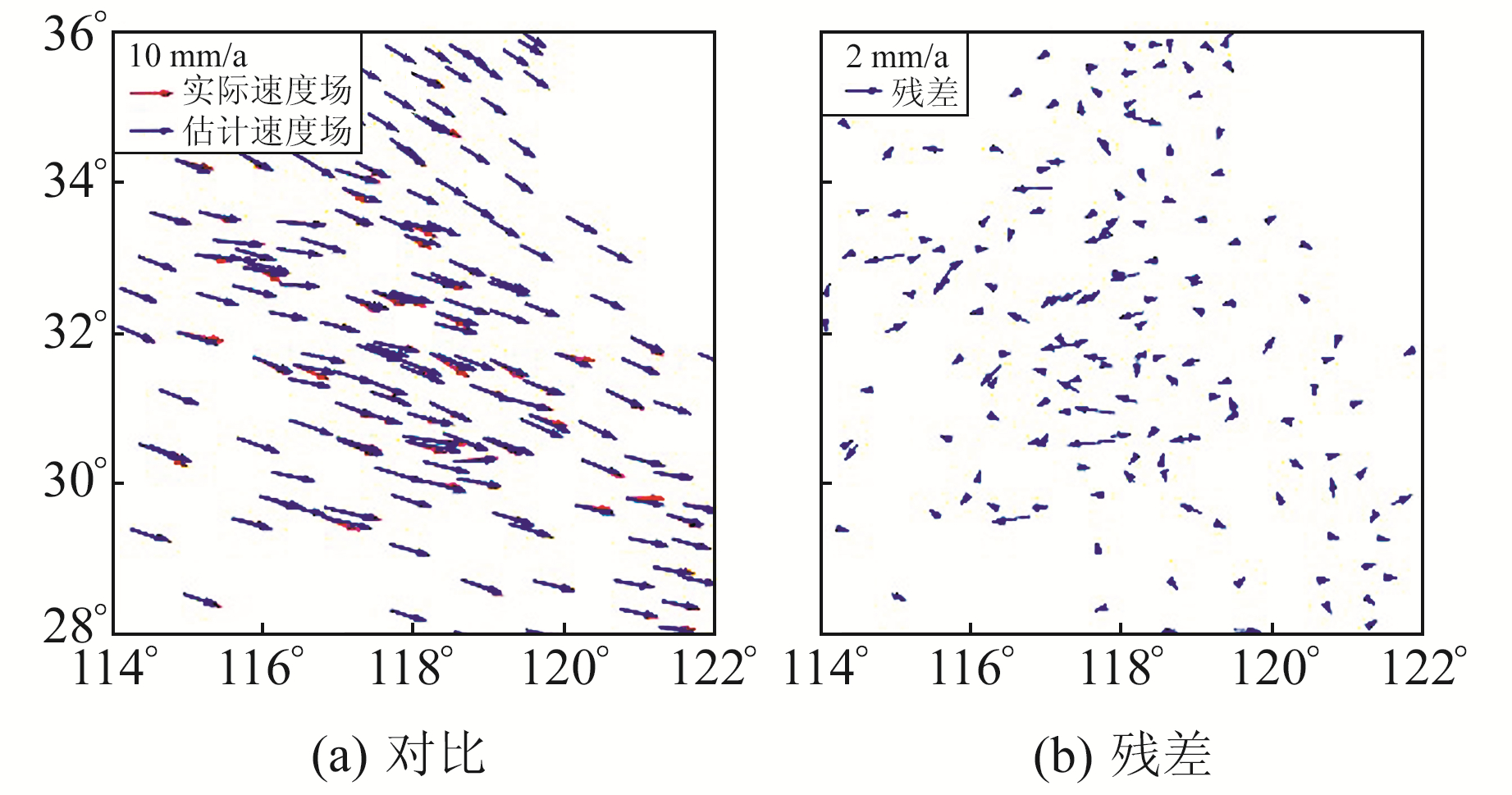图 4 多尺度球面小波估计速度场与实际速度场的矢量对比 Fig. 4 Vector comparison of velocity field estimated by multi-scale spherical wavelet and actual velocity field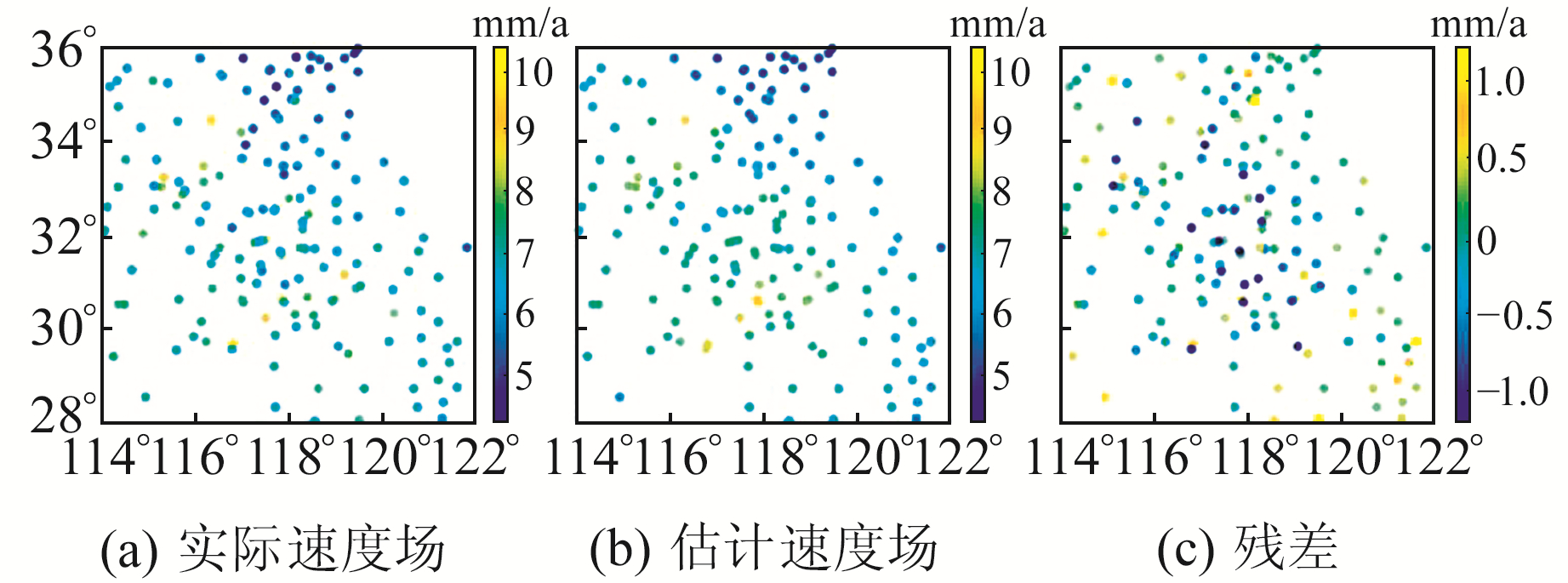图 5 多尺度球面小波估计速度场与实际速度场的标量对比 Fig. 5 Scalar comparison of velocity field estimated by multi-scale spherical wavelet and actual velocity field
2.3 应变场解算

 $\begin{array}{l} \;\;\;\;\;\;\;\;\;\;\;\;\;\;D = \left[ {\begin{array}{*{20}{c}} {{e_{\theta \theta }}}&{{e_{\theta \varphi }}}\\ {{e_{\theta \varphi }}}&{{e_{\varphi \varphi }}} \end{array}} \right] = \\ \left[ {\begin{array}{*{20}{c}} {\frac{1}{r}\cdot\frac{{\partial {u_\theta }}}{{\partial \theta }}}&{\frac{1}{{2r}}\left( {\frac{{\partial {u_\varphi }}}{{\partial \theta }} + \frac{1}{{\sin \theta }}\cdot\frac{{\partial {u_\theta }}}{{\partial \theta }} - {u_\varphi }\cot \theta } \right)}\\ {{D_{23}}}&{\frac{1}{r}\left( {\frac{1}{{\sin \theta }}\cdot\frac{{\partial {u_\varphi }}}{{\partial \varphi }} + {u_\theta }\cot \theta } \right)} \end{array}} \right] \end{array}$ (4)

3 郯庐断裂带南段地壳形变与断层活动分析 3.1 应变场分析图 6 基于多尺度球面小波解算的面膨胀率 Fig. 6 The dilatation rate of multiscale spherical wavelet solution图 7 qmax=8时的主应变率/面膨胀率和最大剪应变率 Fig. 7 Principal strain rate/ dilatation rate and maximum shear strain rate at qmax=8
3.2 速度剖面分析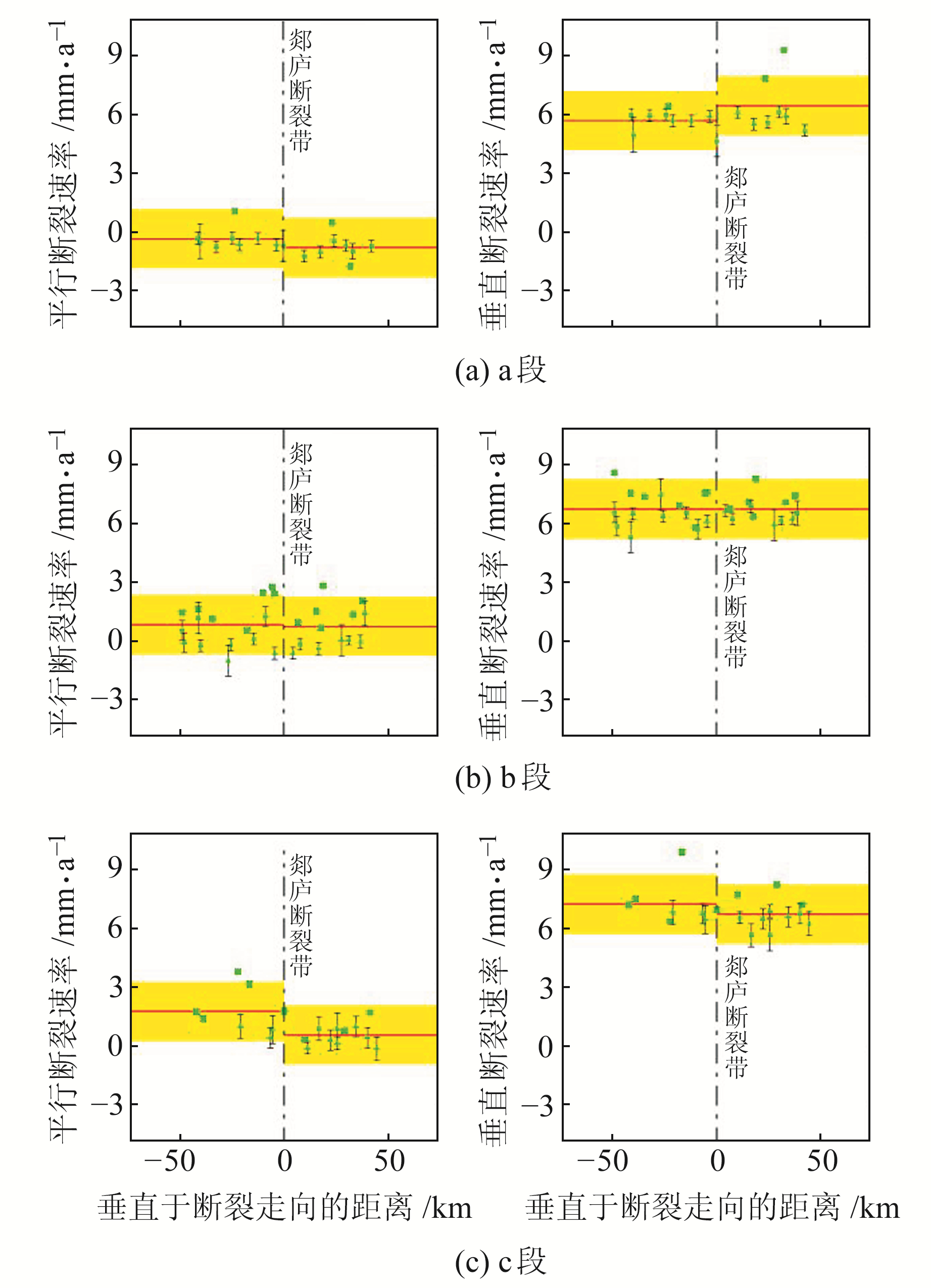图 8 郯庐断裂带南段的3个速度剖面 Fig. 8 Three velocity profiles on the southern segment of the Tanlu fault表 3 郯庐断裂带南段断层滑动速率(右旋拉张为正) Tab. 3 Slip rates on the southern of the Tanlu fault (right-lateral with tension is positive)
4 结语

1) 安徽地区在欧亚框架下主要朝东偏南方向运动。本文利用多尺度球面小波法模拟出的速度场与实际速度场的误差在2 mm/a以内，可有效反映该区域的地壳运动情况。

2) 总体上看，郯庐断裂带南段以西除阜阳、徐州、枣庄等地外，大部分地区呈面膨胀状态，以东地区大多表现为面压缩状态。根据最大分解尺度下的主应变率/面膨胀率和最大剪应变率的分布情况可以看出，应变方向变化异常的地区，均出现在面膨胀与面压缩的交界地带。最大剪应变率沿郯庐断裂带南段两侧呈对称分布，其西北和东南地区为高值区域，构造特征复杂，存在着较大的应变积累，有发生地震的可能，应予以关注。

3) 郯庐断裂带南段主要表现为右旋走滑，且北部存在着拉张，拉张分量向南逐渐递减，在最南部转变为压缩的状态。平行断裂的运动速率为0.10~1.21 mm/a-1，垂直断裂的运动速率为-0.52~0.76 mm/a。

  李腊月, 李玉江, 张风霜, 等. 郯庐断裂带中南段闭锁特征与地震危险性分析[J]. 地质学报, 2020, 94(2): 467-479 (Li Layue, Li Yujiang, Zhang Fengshuang, et al. Fault Blocking Characteristics and Seismic Hazard Analysis in the Middle and Southern Segments of the Tanlu Fault Zone[J]. Acta Geologica Sinica, 2020, 94(2): 467-479 DOI:10.3969/j.issn.0001-5717.2020.02.008) (0)  李彦川, 单新建, 宋小刚, 等. GPS揭示的郯庐断裂带中南段闭锁及滑动亏损[J]. 地球物理学报, 2016, 59(11): 4022-4034 (Li Yanchuan, Shan Xinjian, Song Xiaogang, et al. Fault Locking and Slip Rate Deficit on the Middle and Southern Segment of the Tancheng-Lujiang Fault Inverted from GPS Data[J]. Chinese Journal of Geophysics, 2016, 59(11): 4022-4034 DOI:10.6038/cjg20161108) (0)  Tape C, Musé P, Simons M, et al. Multiscale Estimation of GPS Velocity Fields[J]. Geophysical Journal International, 2009, 179(2): 945-971 DOI:10.1111/j.1365-246X.2009.04337.x (0)  苏小宁, 孟国杰, 王振. 基于多尺度球面小波解算GPS应变场的方法及应用[J]. 地球物理学报, 2016, 59(5): 1585-1595 (Su Xiaoning, Meng Guojie, Wang Zhen. Methodology and Application of GPS Strain Field Estimation Based on Multi-Scale Spherical Wavelet[J]. Chinese Journal of Geophysics, 2016, 59(5): 1585-1595) (0)  徐克科, 伍吉仓. GNSS多尺度应变场估计及区域形变检测[J]. 测绘学报, 2016, 45(8): 992-1000 (Xu Keke, Wu Jicang. Estimation of GNSS Multiscale Strain Field and Detection of Regional Crustal Deformation[J]. Acta Geodaetica et Cartographica Sinica, 2016, 45(8): 992-1000) (0)  李承涛, 苏小宁, 孟国杰. 巴颜喀拉块体东北缘GPS应变率空间分布特征及其与2017年九寨沟MS7.0地震的关系[J]. 地震, 2018, 38(2): 37-50 (Li Chengtao, Su Xiaoning, Meng Guojie. Heterogeneous Strain Rate Field in the Northeast Margin of Bayan Har Block from GPS Observations and Its Relationship with the 2017 Jiuzhaigou MS7.0 Earthquake[J]. Earthquake, 2018, 38(2): 37-50) (0)  Wang M, Shen Z K. Present-Day Crustal Deformation of Continental China Derived from GPS and Its Tectonic Implications[J]. Journal of Geophysical Research: Solid Earth, 2020, 125(2) (0)  袁鹏, 孙宏飞, 秦昌威, 等. 安徽CORS参考站三维速度场分析[J]. 武汉大学学报: 信息科学版, 2016, 41(4): 535-540 (Yuan Peng, Sun Hongfei, Qin Changwei, et al. Analysis of Anhui CORS Reference Stations 3D Velocity Field[J]. Geomatics and Information Science of Wuhan University, 2016, 41(4): 535-540) (0)  Savage J C, Gan W J, Svarc J L. Strain Accumulation and Rotation in the Eastern California Shear Zone[J]. Journal of Geophysical Research: Solid Earth, 2001, 106(B10): 21995-22007 DOI:10.1029/2000JB000127 (0)  孟国杰, 任金卫, 伍吉仓, 等. 球坐标系中图形单元应变与旋转张量及其误差解算[J]. 地震学报, 2008, 30(1): 67-75 (Meng Guojie, Ren Jinwei, Wu Jicang, et al. Computation of Strain and Rotation Tensor and Their Uncertainty for Small Arrays in Spherical Coordinate System[J]. Acta Seismologica Sinica, 2008, 30(1): 67-75 DOI:10.3321/j.issn:0253-3782.2008.01.007) (0)  郭良迁, 薄万举, 杨国华, 等. 华北1999~2009年水平形变应变场特征[J]. 大地测量与地球动力学, 2011, 31(3): 15-19 (Guo Liangqian, Bo Wanju, Yang Guohua, et al. Characteristics of Horizontal Deformation-Strain Field in North China from 1999 to 2009[J]. Journal of Geodesy and Geodynamics, 2011, 31(3): 15-19) (0)  宋传中, 王国强, 朱光, 等. 郯庐断裂带桐城-庐江段的构造特征及演化[J]. 安徽地质, 1998, 8(4): 37-40 (Song Chuanzhong, Wang Guoqiang, Zhu Guang, et al. Tectonic Characteristics and Evolution of Tongcheng-Lujiang Segment of the Tan-Lu Fault Zone[J]. Geology of Anhui, 1998, 8(4): 37-40) (0)
Analysis of Crustal Deformation and Fault Activity Characteristics in the Southern Segment of Tanlu Fault Zone with AHCORS
CHEN Hao1TAO Tingye1LI Shuiping1     LI Jiangyang1     SHI Mengjie1     GAO Fei1
1. College of Civil Engineering, Hefei University of Technology, 193 Tunxi Road, Hefei 230009, China
Abstract: Using GAMIT/GLOBK software, we process the observation files of 37 AHCORS reference stations during Jan. 2013 and June 2018, and obtain the velocity fields of the stations under ITRF2008 and Eurasia frameworks. The error between the velocity field simulated by multi-scale spherical wavelet and the actual velocity field is less than 2 mm/a, indicating that this method effectively reflects crustal movement in this region. We use multiscale spherical wavelet to calculate and analyze the strain rates of the southern segment of the Tanlu fault zone and its surrounding areas. The results show that most of the areas west of the southern segment of the Tanlu fault zone are compressed, while most of the areas east of the Tanlu fault zone are dilatated in general. The abnormal variation of strain direction is located at the junction of plane expansion and plane compression. The maximum shear strain rate is symmetrically distributed along both sides of the southern segment of the Tanlu fault zone, and the northwest and southeast regions are high value regions with large strain accumulation and the possibility of earthquake, to which more attention should be paid. The southern segment of the Tanlu fault zone is mainly dextral strike-slip, there is tension in the north, the tensile component gradually decreases in the south, and the southernmost part turns into compression state.
Key words: AHCORS; velocity field; spherical wavelet; strain; velocity profile